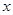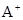# NCERT Solutions class-11 Maths Exercise 14.4## myCBSEguide App

CBSE, NCERT, JEE Main, NEET-UG, NDA, Exam Papers, Question Bank, NCERT Solutions, Exemplars, Revision Notes, Free Videos, MCQ Tests & more.

Exercise 14.4

1. Rewrite the following statements with “if-then” in five different ways conveying the same meaning.

If a natural number is off then its square is also odd.

Ans. (i) A natural number is odd implies that its square is odd.

(ii) A natural is odd only if its square is odd.

(iii) For a natural number to be odd it is necessary that its square is odd.

(iv) For the square of a natural number to be odd it is sufficient that the number is odd.

(v) If the square of a natural number is not odd then the natural number is not odd.

2. Write the contrapositive and converse of the following statements:

(i) Ifis a prime number, thenis odd.

(ii) If the two lines are parallel, then they do not intersect in the same plane.

(iii) Something is cold implies that is has low temperature.

(iv) You cannot comprehend geometry if you do not know how to reason deductively.

(v)is an even number implies thatis divisible by 4.

Ans. (i) The contrapositive of given statement is: Ifis not odd thenis not a prime number.

The converse of the given statement is: Ifis an odd number thenis a prime number.

(ii) The contrapositive of given statement is: If two lines intersect in the same plane then they are not parallel.

The converse of the given statement is: If the two lines do not intersect in the same plane they are parallel.

(iii) The contrapositive of given statement is: If something does not have low temperature then it is not cold.

The converse of the given statement is: If something has low temperature then it is cold.

(iv) The contrapositive of given statement is: If you know to reason deductively then you can comprehend geometry.

The converse of the given statement is: If you do not know to reason deductively then you cannot comprehend geometry.

(v) The contrapositive of given statement is: Ifis not divisible by 4 thenis not an even number.

The converse of the given statement is: Ifis divisible by 4 thenis an even number.

3. Write each of the following statements in the form “if-then”:

(i) You get a job implies that your credentials are good.

(ii) The Banana tree will bloom if it stays warm for a month.

(iii) A quadrilateral is a parallelogram, if its diagonals bisect each other.

(iv) To get an A+ in the class then you do all the exercises of the book.

Ans. (i) If you get a job then you credentials are good.

(ii) If it says warm for a month then the banana trees will bloom.

(iii) If the diagonals of a quadrilateral bisect each other then it is a parallelogram.

(iv) If you getin the class then you do all the exercises in the book.

4. Give statements in (a) and (b). Identify the statements given below as contrapositive or converse of each other:

(a) If you live in Delhi, then you have winter clothes.

(i) If you do not have winter clothes, then you do not live in Delhi.

(ii) If you have winter clothes, then you live in Delhi.

(b) If a quadrilateral is a parallelogram then its diagonals bisect each other.

(i) If the diagonals of a quadrilateral do not bisect each other then the quadrilateral is not a parallelogram.

(ii) If the diagonals of a quadrilateral bisect each other then the quadrilateral is a parallelogram.

Ans. (a) (i) Contrapositive (ii) Converse

(b) (i) Contrapositive (ii) Converse